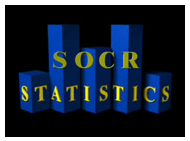# Statistics 100B: Introduction to Mathematical Statistics

### Announcements

• First lecture is on Monday, 09 January 2023
Location: Dodd 175
Day/time: MWF 14:00 - 14:50
See you then!

• http://www.socr.ucla.edu

####• http://wiki.stat.ucla.edu/socr/index.php/SOCR_EduMaterials

### Handouts

• 1. Summary of discrete and continuous distributions (review from Statistics 100A).
• The next five links are the statistical tables needed for the entire quarter (from "Mathematical Statistics and Data Analysis", by John Rice, Doxbury Press, Second Edition (1995)).
Table 1: Standard normal distribution table (Z).
Table 2: Chi-square distribution table.
Table 3: t distribution table.
Table 4: F distribution table (95th percentiles).
Table 5: F distribution table (99th percentiles).
• 2. Some review material from Statistics 100A.
• 3. Simulation of random variables.
• 4. Covariance and correlation.
• 5. Poisson, Exponential, and Gamma distributions.